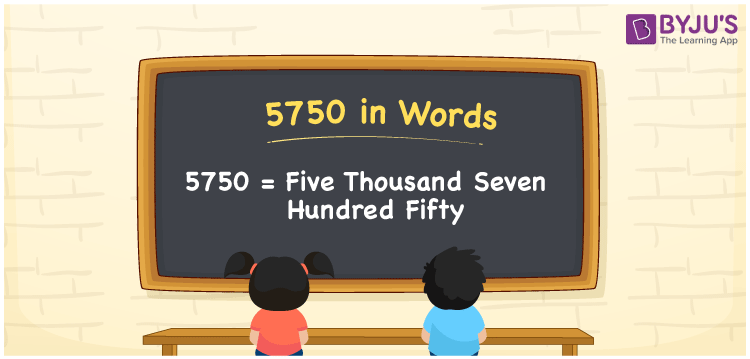# 5750 in Words

We can write 5750 in words as Five thousand seven hundred fifty. When we are asked to read or spell the number 5750, we use the words “Five thousand seven hundred fifty”. If this number represents the cost of a certain thing, we may call it “Five thousand seven hundred fifty rupees”. Also, when the number 5750 denotes the weight of a rice packet in grams, we spell the same as “The weight of the rice packet is Five thousand seven hundred fifty grams”. Thus, we use the word form of the cardinal number in different contexts.

 5750 in words Five thousand seven hundred fifty Five thousand seven hundred fifty in Numbers 5750

## 5750 in English Words

Using the English alphabet, we generally write numbers in words. So, we can read 5750 in words as “Five thousand seven hundred fifty.## How to Write 5750 in Words?

We can convert the number 5750 into words using a four-column place value chart since it has four digits. Thus, we need to write the place value for each of these four digits as shown below.

 Thousands Hundreds Tens Ones 5 7 5 0

Here, ones = 0, tens = 5, hundreds = 7, and thousands = 5.

Thus, we can write the expanded form as:

5 × Thousand + 7 × Hundred + 5 × Ten + 0 × One

= 5 × 1000 + 7 × 100 + 5 × 10 + 0 × 1

= 5000 + 700 + 50

= Five thousand + Seven hundred + Fifty

= Five thousand seven hundred fifty

Hence, 5750 in words = Five thousand seven hundred fifty.

5750 is a natural number that precedes 5751 and succeeds 5749.

5750 in words – Five thousand seven hundred fifty

Is 5750 an odd number? – No

Is 5750 an even number? – Yes

Is 5750 a prime number? – No

Is 5750 a composite number? – Yes

Is 5750 a perfect square number? – No

Is 5750 a perfect cube number? – No

## Frequently Asked Questions on 5750 in Words

Q1

### How do you write 5750 in words?

The number 5750 can be written in words as Five thousand seven hundred fifty.
Q2

### How to write Rs. 5750 in words on a cheque?

On a cheque, we should write Rs. 5750 in words as Five thousand seven hundred fifty rupees only.
Q3

### Write the value of 5750 – 4025 in words

5750 – 4025 = 1725 Therefore, the value of 5750 – 4025 is 1725.